Test: Number Representations- 1

# Test: Number Representations- 1

Test Description

## 20 Questions MCQ Test RRB JE for Computer Science Engineering | Test: Number Representations- 1

Test: Number Representations- 1 for Computer Science Engineering (CSE) 2022 is part of RRB JE for Computer Science Engineering preparation. The Test: Number Representations- 1 questions and answers have been prepared according to the Computer Science Engineering (CSE) exam syllabus.The Test: Number Representations- 1 MCQs are made for Computer Science Engineering (CSE) 2022 Exam. Find important definitions, questions, notes, meanings, examples, exercises, MCQs and online tests for Test: Number Representations- 1 below.
Solutions of Test: Number Representations- 1 questions in English are available as part of our RRB JE for Computer Science Engineering for Computer Science Engineering (CSE) & Test: Number Representations- 1 solutions in Hindi for RRB JE for Computer Science Engineering course. Download more important topics, notes, lectures and mock test series for Computer Science Engineering (CSE) Exam by signing up for free. Attempt Test: Number Representations- 1 | 20 questions in 60 minutes | Mock test for Computer Science Engineering (CSE) preparation | Free important questions MCQ to study RRB JE for Computer Science Engineering for Computer Science Engineering (CSE) Exam | Download free PDF with solutions
 1 Crore+ students have signed up on EduRev. Have you?
*Answer can only contain numeric values
Test: Number Representations- 1 - Question 1

### The 16  -bit 2` s complement representation of an integer is 1111  1111 1111    0101 ; its decimal representation is ______________.

Detailed Solution for Test: Number Representations- 1 - Question 1

for 2's complement  1 111 1111 1111 0101 →2′scomplement→2′scomplement  1 000 0000 0000 1011
1st bit is same not involved in 2's complement same with 1's complement. since msb bit for sign.
Take one's complement and add 1 we get 11, and as it is negative number we get answer as -11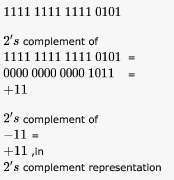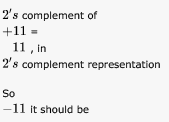*Answer can only contain numeric values
Test: Number Representations- 1 - Question 2

### Let X be the number of distinct 16-bit integers in 2`s complement representation. Let Y be the number of distinct -bit integers in sign magnitude representation Then X  Y is______.

Detailed Solution for Test: Number Representations- 1 - Question 2

For N bits, Distinct values represented in 2's complement is -2n-1 to 2n-1 -1
Distinct values represented in Signed Magnitude is -(2n-1 -1) to 2n-1 -1
Difference is 1.

*Answer can only contain numeric values
Test: Number Representations- 1 - Question 3

### Define the value of r in the following: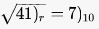Detailed Solution for Test: Number Representations- 1 - Question 3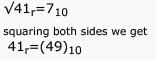4r+1=40+9
4r=48
r=12

*Answer can only contain numeric values
Test: Number Representations- 1 - Question 4

Consider the number given by the decimal expression: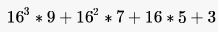The number of 1’s in the unsigned binary representation of the number is ______

Detailed Solution for Test: Number Representations- 1 - Question 4

Hex representation of given no. is (9753)16
Its binary representation is (1001 0111 0101 0011)2 The no. of 1's is 9

*Answer can only contain numeric values
Test: Number Representations- 1 - Question 5

Convert the following numbers in the given bases into their equivalents in the desired bases:

a. (110.101)2 = (x)10
b. (1118)10 = (y)H

Detailed Solution for Test: Number Representations- 1 - Question 5

a. 1 * 22 + 1 * 21 + 0 * 20 + 1 * 2-1 + 0 * 2-2 + 1 * 2-3 = 6.625
b. 1118 mod 16 = 14, quotient = 69
69 mod 16 = 5, quotient = 4
4 mod 16 = 4.
Writing the mods in the reverse order (in hex) gives (45E)H
Both can be done using calculator also.

Test: Number Representations- 1 - Question 6

The number of 1's in the binary representation of (3*4096 + 15*256 + 5*16 + 3) are:

Detailed Solution for Test: Number Representations- 1 - Question 6

I suggest following approach , here we can clearly see that numbers are getting multiplied by powers of 16. So this is nothing but Hexadecimal number in disguise.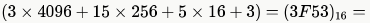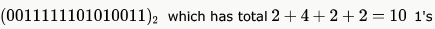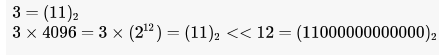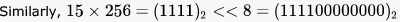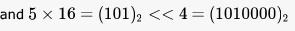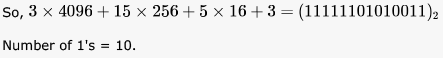Test: Number Representations- 1 - Question 7

Consider the following floating-point number representation.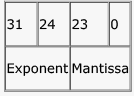The exponent is in 2’s complement representation and mantissa is in the sign magnitude representation. The range of the magnitude of the normalized numbers in this representation is

Detailed Solution for Test: Number Representations- 1 - Question 7

Here, we are asked "magnitude" - so we just need to consider the mantissa bits.
Also, we are told "normalized representation"- so most significant bit of mantissa is always 1 (this is different from IEEE 754 normalized representation where this 1 is omitted in representation, but here it seems to be added on the right of decimal point as seen from options).
So, the maximum value of mantissa will be 23 1's where a decimal point is assumed before first 1. So, this value will be 1- 2-23.
Due to the 1 in normalized representation, the smallest positive number will be 1 followed by 23 0's which will be 2-1 = 0.5

Given that mantissa is sign magnitude representation so 1 bit for sign and remaining 23 bits for mantissa and  we have to find the range of mantissa in normalized form ....
so smallest will be = .100.....0(normalize form) which is 2^(-1) = .5
and for largest will be .111....1 which is 1-2^(-23) .
so range will be .5 to (1-2^(-23))

Test: Number Representations- 1 - Question 8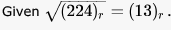The value of the radix r is:

Detailed Solution for Test: Number Representations- 1 - Question 8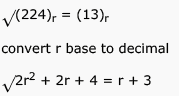2r2 + 2r + 4 = r2 + 6r+ 9
r2 - 4r -5 = 0
r2 -5r +r - 5 = 0
(r-5)(r+1)=0
r can not be -1
so r = 5 is correct answer

Test: Number Representations- 1 - Question 9

The octal representation of an integer is (342)8. If this were to be treated as an eight-bit integer in an 8085 based computer, its decimal equivalent is

Detailed Solution for Test: Number Representations- 1 - Question 9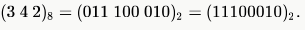If we treat this as an 8 bit integer, the first bit becomes sign bit and since it is "1", number is negative. Computer uses 2's complement representation for negative numbers and hence the decimal equivalent will be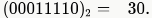Test: Number Representations- 1 - Question 10

Suppose the domain set of an attribute consists of signed four digit numbers. What is the percentage of reduction in storage space of this attribute if it is stored as an integer rather than in character form?

Detailed Solution for Test: Number Representations- 1 - Question 10

I assume byte addressable memory- nothing smaller than a byte can be used.
We have four digits. So, to represent signed 4 digit numbers we need 5 bytes- 4 for four digits and 1 for the sign (like -7354). So, required memory = 5 bytes
Now, if we use integer, the largest number needed to represent is 9999 and this requires 2 bytes of memory for signed representation.

So, memory savings while using integer is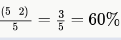*Multiple options can be correct
Test: Number Representations- 1 - Question 11

Zero has two representations in

Detailed Solution for Test: Number Representations- 1 - Question 11

Sign Magnitude
+0 = 0000
-0 = 1000
1's complement
+0 = 0000
-0 = 1111

Test: Number Representations- 1 - Question 12

The number 43 in 2's complement representation is

Detailed Solution for Test: Number Representations- 1 - Question 12

2's complement representation is not same as 2's complement of a number. In 2's complement representation positive integers are represented in its normal binary form while negative numbers are represented in its 2's complement form. So (c) is correct here.

Test: Number Representations- 1 - Question 13

Consider the values of A = 2.0 x 1030, B = -2.0 x 1030 , C = 1.0, and the sequence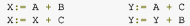executed on a computer where floating point numbers are represented with 32 bits. The values for X and Y will be

Detailed Solution for Test: Number Representations- 1 - Question 13

Given 32 bits representation. So, the maximum precision can be 32 bits (In 32-bit IEEE representation, maximum precision is 24 bits but we take best case here). This means approximately 10 digits.
A = 2.0 * 1030, C = 1.0
So, A + C should make the 31st digit to 1, which is surely outside the precision level of A (it is 31st digit and not 31st bit).
So, this addition will just return the value of A which will be assigned to Y. So, Y + B will return 0.0 while X + C will return 1.0.
B choice.

Test: Number Representations- 1 - Question 14

The 2's complement representation of (-539)10 in hexadecimal is

Detailed Solution for Test: Number Representations- 1 - Question 14

539 = 512 + 16 + 8 + 2 + 1 = 29 + 24 + 23 + 21 + 20
= (1000011011)2
Now all answers have 12 bits, so we add two 0's at beginning = (001000011011)2
To convert to 2's complement invert all bits till the rightmost 1, which will be (110111100101)2
= (1101 1110 0101)2
= (DE5)16

Test: Number Representations- 1 - Question 15

The decimal value 0.25

Detailed Solution for Test: Number Representations- 1 - Question 15

1st Multiplication Iteration Multiply 0.25 by 2
0.25 x 2 = 0.50(Product)         Fractional part=0.50
Carry=0    (MSB)
2nd Multiplication Iteration
Multiply 0.50 by 2
0.50 x 2 = 1.00(Product)         Fractional part = 1.00
Carry = 1(LSB)
The fractional part in the 2nd iteration becomes zero and hence we stop the multiplication iteration.
Carry from the 1st multiplication iteration becomes MSB and  carry from 2nd iteration becomes LSB
So the Result is 0.01

Test: Number Representations- 1 - Question 16

The 2's complement representation of the decimal value -15 is

Detailed Solution for Test: Number Representations- 1 - Question 16

is the correct ans.In 2's complement representation, positive numbers are represented in simple binary form and negative numbers are represented in its 2's complement form. So, for -15, we have to complement its binary value -01111 and add a 1 to it, which gives 10001. Option D.

Test: Number Representations- 1 - Question 17

Sign extension is a step in

Detailed Solution for Test: Number Representations- 1 - Question 17

is the answer. Sign extension (filling the upper bits using the sign bit) is needed while increasing the number of bits for representing a number. For positive numbers, 0 is extended and for negative numbers 1 is extended.

Test: Number Representations- 1 - Question 18

Detailed Solution for Test: Number Representations- 1 - Question 18

is the answer. When a positive value and negative value are added overflow never happens.

Test: Number Representations- 1 - Question 19

Assuming all numbers are in 2’s complement representation, which of the following numbers is divisible by 11111011?

Detailed Solution for Test: Number Representations- 1 - Question 19

MSB of 2's compliment number has a weight of - 2^(n-1).
(Trick: (from reversing sign extension) just skip all leading 1's from MSB expect but 1, and then calculate the value as normal signed binary rep. ) so by calculating, we get the given number is -5 in decimal. and options are
a. -25
b. -28
c. -41
d. -37
Therefore it is clear that - 25 is divisible by - 5. so we can say that (a.) is correct.

Test: Number Representations- 1 - Question 20

If 73x (in base-x number system) is equal to 54y (in base y-number system), the possible values of x and y are

Detailed Solution for Test: Number Representations- 1 - Question 20

x * 7 + 3 = 5 * y + 4 => 7x = 5y + 1. Only option satisfying this is D.

## RRB JE for Computer Science Engineering

3 videos|7 docs|100 tests
 Use Code STAYHOME200 and get INR 200 additional OFF Use Coupon Code
Information about Test: Number Representations- 1 Page
In this test you can find the Exam questions for Test: Number Representations- 1 solved & explained in the simplest way possible. Besides giving Questions and answers for Test: Number Representations- 1, EduRev gives you an ample number of Online tests for practice

## RRB JE for Computer Science Engineering

3 videos|7 docs|100 tests